Next: Curvilinear coordinates Up: Useful mathematics Previous: Rotational coordinate transformations

# Precession

Suppose that some position vector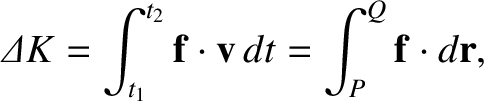precesses (i.e., rotates) about the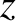-axis at the angular velocity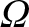. If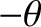,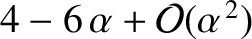,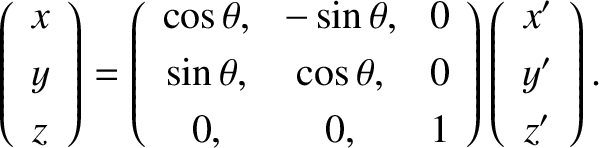are the Cartesian components ofat time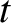then it follows from the analysis in the previous section that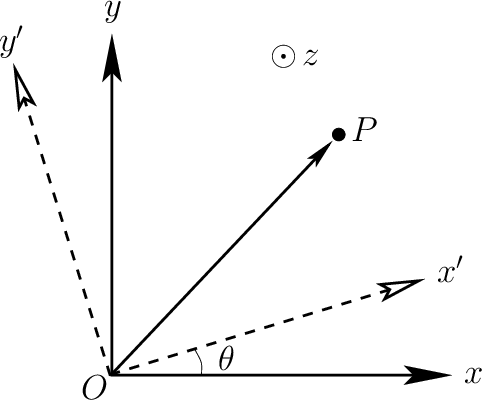(A.93)

Hence, making use of the small angle approximations to the sine and cosine functions, we obtain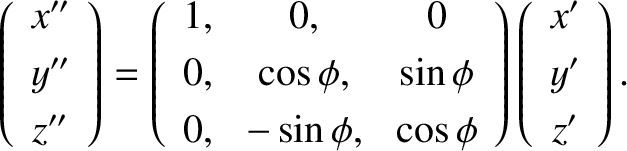(A.94)

which immediately implies that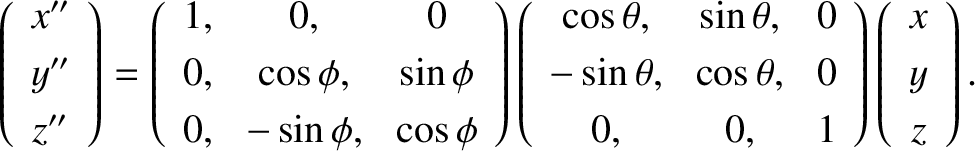(A.95)

or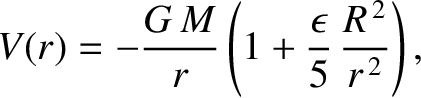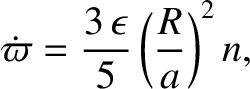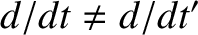(A.96)

where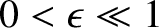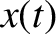is the angular velocity of precession. Because vector equations are coordinate independent, we deduce that the preceding expression is the general equation for the time evolution of a position vectorthat precesses at the angular velocity.Next: Curvilinear coordinates Up: Useful mathematics Previous: Rotational coordinate transformations
Richard Fitzpatrick 2016-03-31